## Cost management system

### This system can be linked with Simple Schedule Editor

•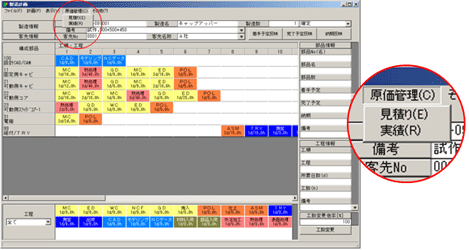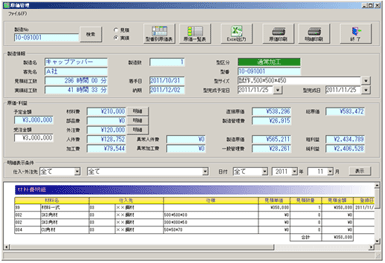•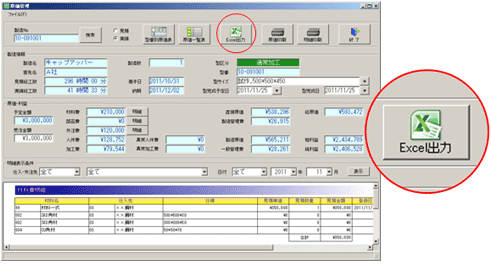• After formulating a plan, you can immediately obtain cost estimate table of each mould.
• After inputting results on the field, you can obtain actual cost calculation table of each mould.
• By means of Excell output and linking function, you can validate the cost calculation tables.

### Formulation of various cost tables and Excel output

•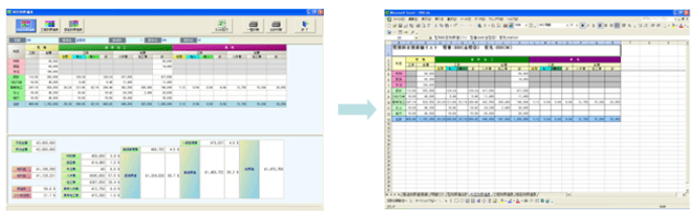• Cost table of each item

•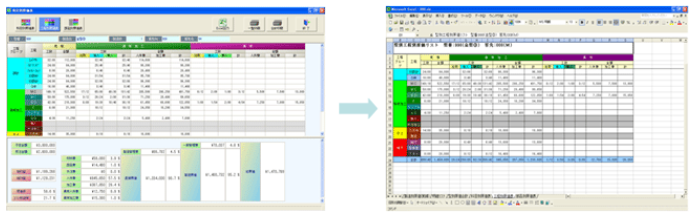• Cost table of each procedure

•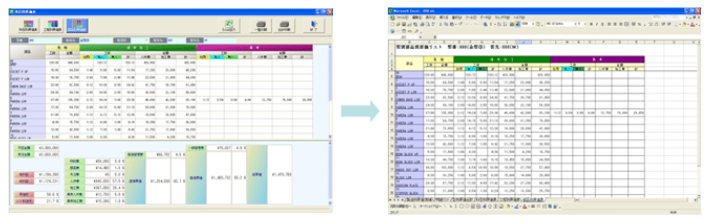• Cost table of each part

•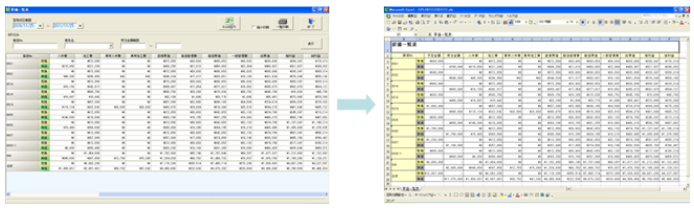• Cost list

• Formulation of cost table of each item, cost table of each procedure and cost table of each part.
• Formulation of cost list for specified period.
• The outputs can be saved as excel file.